• matlab中x=X(1:N)是什么意思：1:5 表示是 (1,2,3,4,5) 这样一个向量 。X(1:N) 当然就是取X中前N个元素了.。 例如，我想在1-60之间得到10个服从标准正态分布的随机数，如何实现？？ 先用标准正态随机产生函数randn...
matlab中x=X(1:N)是什么意思： 1:5 表示是 (1,2,3,4,5) 这样一个向量 。X(1:N) 当然就是取X中前N个元素了.。
例如，我想在1-60之间得到10个服从标准正态分布的随机数，如何实现？？
先用标准正态随机产生函数randn（1，n);生产一个符合标准正态分布的数列，看看其最大最小值是多少，然后在函数前应映的乘以某个常数来达到你想要求的定义域范围。你想要的是1~60，那需要乘以10以上的常数了，randn(1,100000),产生的数才到4.3左右，例子：x=23.*randn(1,500);  %产生500个元素，          y=find(x>=1&x<=60);% 找出大于1且小于60的元素。y数组为x大于1小于60的元素的位置序号，别搞错了          x=x(y(1:10));%找出这些位置所对应的x的值，找出10个。

转载于:https://www.cnblogs.com/vinn/p/8781639.html
展开全文• 1 randi()函数生成均匀分布的伪随机整数，范围为imin--imax，如果没指定imin，则默认为1。 r = randi(imax,n):生成n*n的矩阵 r = randi(imax,m,n)：生成m*n的矩阵 r = randi(imax,[m,n]):同上 r = randi(imax,m...
1 randi()函数生成均匀分布的伪随机整数，范围为imin--imax，如果没指定imin，则默认为1。

r = randi(imax,n):生成n*n的矩阵

r = randi(imax,m,n)：生成m*n的矩阵

r = randi(imax,[m,n]):同上

r = randi(imax,m,n,p,...)：生成m*n*p*...的矩阵

r = randi(imax,[m,n,p,...])同上

r = randi(imax)：1*1的矩阵

r = randi(imax,size(A))：和size(A）同维的矩阵

r = randi([imin,imax],...)

2rand ()函数生成0-1的矩阵

r = rand(a,b):生成a*b的0-1(1以内)的矩阵

r = rand(3)：生成3*3的0-1(1以内)的矩阵

r = rand(size(A))：和size(A）同维的矩阵

r = rand(a，b,'single/double');指定精度的矩阵

r = rand(RandSteam,a,b);Rand Steam要生成的限制，生成什么样子的种子。a*b

3randn（）函数,生成的是均值为0方差为1的正态分布形状的矩阵


展开全文• 在用matlab进行编程时经常会混淆这三个函数的用法，下面通过例子简明说明一下每个函数的用法。 rand()函数是产生（0,1）区间的任意随机数，有范围限制，如果写成rand(m,n)的形式就是产生m行n列的随机项矩阵，并且...
在用matlab进行编程时经常会混淆这三个函数的用法，下面通过例子简明说明一下每个函数的用法。
rand()函数是产生（0,1）区间的任意随机数，有范围限制，如果写成rand(m,n)的形式就是产生m行n列的随机项矩阵，并且矩阵的每个元素的取值范围都位于（0,1）区间之内。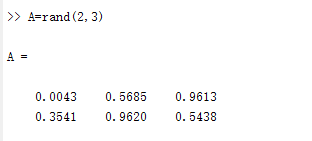如果只产生一个（0,1）区间的随机数的话，rand()函数加不加括号都行。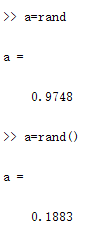randn()函数跟rand()的区别就是没有范围的限制，写成randn(m,n)的形式是产生m行n列的随机项矩阵。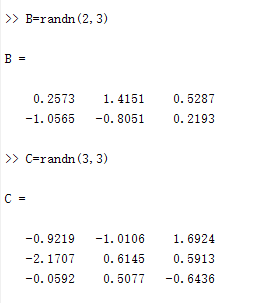randperm(N)函数是产生N个数，这N个数是1~N的N个数，并且顺序打乱，其中还有第二个参数，第二个参数指定个数，如果不指定默认产生N个数。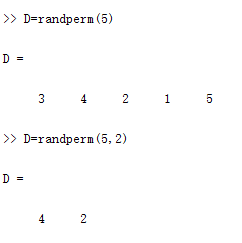展开全文• 1. 2、rand(n):产生范围为0-1的nxn随机数矩阵 1.3、rand(m,n):产生范围为0-1的mxn随机数矩阵 1.4、rand(size(A)):产生范围为0-1，且行列数与A矩阵相同的随机数矩阵。 II.randn用法 2.1、randn:随机的数，服从标准...
• 一、rand()：产生（0,1）之间均匀分布的伪随机数。... x = rand(n)： 返回一个n*n的矩阵，矩阵中所有元素都在（0,1）的范围内。 3. x = rand(n,m) ：返回一个n*m的矩阵，矩阵中所有的元素都在（0,1）的...
一、rand()：产生（0,1）之间均匀分布的伪随机数。  关于均匀分布请戳（待补充）。

语法：

1.  x = rand()   ：返回一个（0,1）之间的伪随机数。如下图：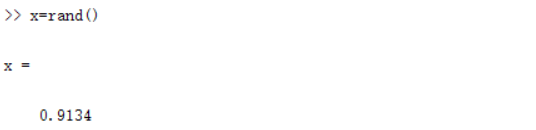2. x = rand(n)： 返回一个n*n的矩阵，矩阵中所有元素都在（0,1）的范围内。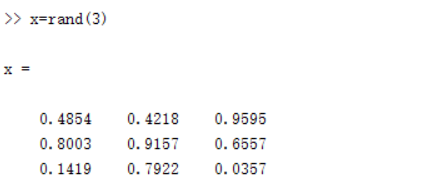3. x = rand(n,m) ：返回一个n*m的矩阵，矩阵中所有的元素都在（0,1）的范围内。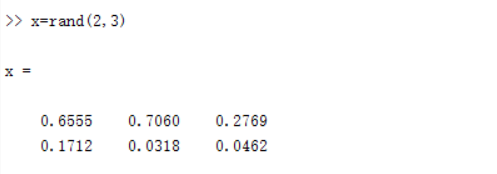二：randn()：产生符合标准正态分布（期望为0，标准差为1）的伪随机数  关于标准正态分布请戳（待补充）

语法应用同rand()一样。

三：randi()：产生一个均匀分布矩阵，矩阵中所有元素都是属于（0,imax]之间的整数。

语法：

1. x = randi(imax) ：产生一个位于（0,imax]之间的整数。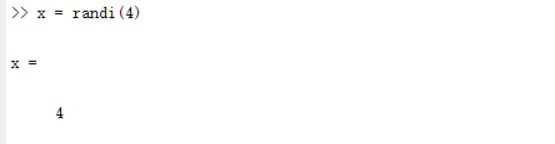2. x = randi(imax,n,m)：产生一个位于（0,imax]之间的n*m的矩阵，所有元素都是整数。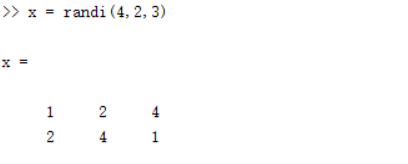3. x = randi([imin,imax],n,m)：产生一个位于[imin,imax]之间的n*m的矩阵，所有的矩阵元素都是整数。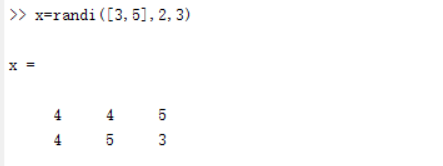最后总结下关于randn('seed',sd)的用法：

对于在产生随机数之前调用randn('seed',sd)，如果sd的值一样，则会产生相同的伪随机数矩阵。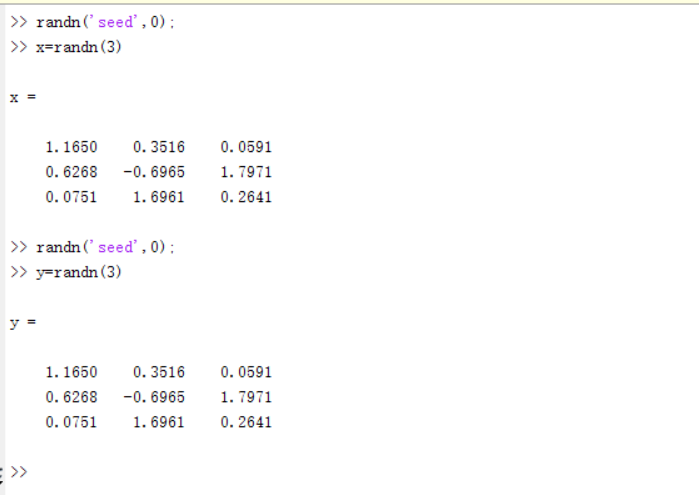当sd的值不同时，则产生不同的伪随机数矩阵。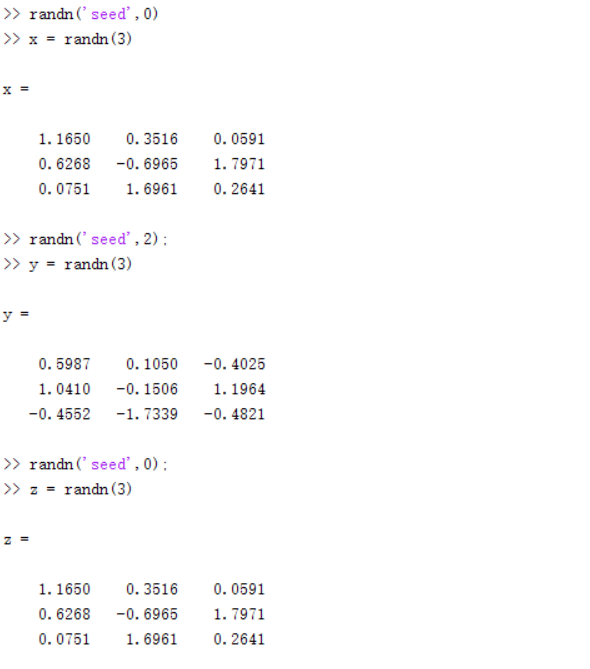展开全文• MATLAB常用语句[持续更新~] Matlab下面几个rand函数，其中： 转：rand(m,n)表示产生m*n的随机...randn(m,n)表示为m*n类型的正态分布随机数矩阵，均值为0，方差为1 randi(max,m,n) 产生值域范围为（0，max）区间内...
• Matlab产生随机数在有些时候是非常必要的，比如在产生某个范围的随机数进行相关的测试等； 函数命令介绍： 1、rand: rand(n):产生0到1之间的n阶随机数方阵； rand(m,n):产生0到1之间的m行n列的随机数矩阵； 2、...
• ## matlab画直方图

千次阅读 2018-03-09 20:45:00
matlab中的数值分析包括了直方图绘制，这里主要介绍hist和histfit命令hist是直接绘制直方图，我们首先给出一个随机数组：aa=randn(1000,1);hist(aa);%当然可以设定直方图内条形的数量：hist(aa,20);横坐标为数值的...
• 1.常用的特殊矩阵包括zeros, ones,eye,rand, randn分别表示全0矩阵，全1矩阵，单位矩阵 ,(0,1)范围均匀分布矩阵，均值为0方差为1的标准正态分布矩阵。 zeros(m)产生的为方阵， zeros(m,n)m*n阶矩阵 zeros(size(A...
• rand(m,n) 生成范围(0,1)的随机矩阵 randperm(n) 生成1~n的不重复随机序列（行向量） randn(m,n) 生成符合正态分布的随机矩阵random
• matlab-rand()随机函数用法 ...randn(m,n) 表示为m*n类型的正态分布随机数矩阵，均值为0，方差为1 rani（max,m,n） 产生值域范围为(0，ma]区间内的随机矩阵。 补充 rand(n) 表示生成一个n阶随机...
• MATLAB中rand()函数表示随机生成大小在0-1范围内的随机数，其参数表示生成随机的行列数。 例如：输入 rand(2，2) 输出 ans=0.2750 0.3232 0.3434 0.5683 randi(x,m,n)函数表示生成最大值为x的m*n矩...
• MATLAB的矩阵生成函数 函数 说明 zeros 产生元素全为0的矩阵 ones 产生元素全为1的矩阵 eye 产生单位矩阵 rand 产生均匀分布的随机数矩阵，数值范围(0,1) randn 产生均值为0，方差为1的正态分布随机数...函数
• rand(m,n)：生成m行n列在 [0,1] 之间满足均匀分布的伪随机数...randn (m,n)：生成m行n列标准正态分布N(0,1) 的伪随机数 小贴士：     要想生成指定范围的均匀分布随机数怎么办？当然是直接乘个系数就OK啊！ ...
• 设计的一个FIR滤波器，对被噪声干扰的信号提取其基波工频信号。...x=sin(2*pi*50*t)+0.7*sin(2*pi*150*t)+0.5*sin(2*pi*250*t)+0.1*randn(size(t)) 设计参数： 窗函数FIR带通滤波器 通带范围：40Hz—60Hz。
• %简单介绍inpolygon函数的用法 ... %创建一个-1~1的范围的点数组 xv = [xv ; xv(1)]; yv = [yv ; yv(1)];%在原来数组基础上加上开始点，从而实现了首尾相连的多边形。 x = randn(250,1); y = randn(250,1
• 随机数的生成在算法编程中非常常用，在matlab中rand(random的缩写)家族经常被使用。 小白一枚，敬请大神指教 rand家族成员 randMatrix = rand(x, y) randMatrix = randn(x, y) randMatrix = randi(x, y) randMatrix...
• 在数理统计中，会经常使用到频率分布直方图，能够直观的反应频率分布的范围大小，在直角坐标系中，横轴为统计变量取值，横轴上的每个小区间对应一个组的组距，作为小矩形的底边；纵轴表示频率与组距的比值，并用它作...
• [(0,1)均匀分布] X = rand(sz1,...,szN)返回由随机数组成的sz1×...×szN数组，其中sz1,...,szN指示每个维度的大小。例如：rand(3,4)返回一个 3×4 的矩阵。 [(a,b)均匀分布] X = unifrnd(a,b,m,n) ...X = randn(...
• 加入高斯白噪声并作平滑处理 clear,clc %% (1) 读取数据 A = imread('LenaRGB....white_noise = 0 + 0.1*randn(m,n); % 白噪声 C = B + white_noise; % 加白噪声 D = C*255; %将像素范围扩大至0--255 D2 = uint8(D);中值滤波
• 课后习题解答 转发请注明出处： 1. 写出完成下列操作的命令。 （1）建立3阶单位矩阵A。 A = eye(3); （2）建立5*6随机矩阵A，其元素为[100,200]范围内的随机整数。...A = 1 + sqrt(0.2)*randn(1, 5
• 如果图片的数据格式为 uint8，也即数据的范围为 [0, 255]，那么直接生成对应方差的噪声，然后加到图片上去。# clean_image uint8 (128, 128) noise_image = clean_image + np.random.randn(128,...
• exp(j*2*pi*randn(N_array,N_snap))/snr;%噪声 SS=data+n; SS_FFT = zeros(N_array,N_snap*N_snap); for seg=1:N_snap %数据段 for m = 1:N_array SS_FFT(m,N_snap*(seg-1)+1:N_...python c++ c语言
• matlab产生一定范围的随机整数和正太分布数 1.函数rand(x)或者rand(x,y)产生0~1之间均匀分布的随机矩阵 2. 要产生1-31范围的100个随机整数 1+round(（31-1）rand(100，1）); 3. round函数用于舍入到最接近的整数。...
• MATLAB中绘制直方图的函数是hist，用法是hist（y，x），表示以向量x的各个元素为统计范围，绘制y的分布情况。具体的用法会非常灵活，我们看下面的教程： 首先我们输入两个变量，x=randn(500,1);y=randn(500...# matlabrandn范围matlab 订阅